Match the vector field F with the correct plot.F(x,\ y)=(x,\ -y)kdgg0909gn 2021-11-20 Answered

Match the vector field F with the correct plot.
$$\displaystyle{F}{\left({x},\ {y}\right)}={\left({x},\ -{y}\right)}$$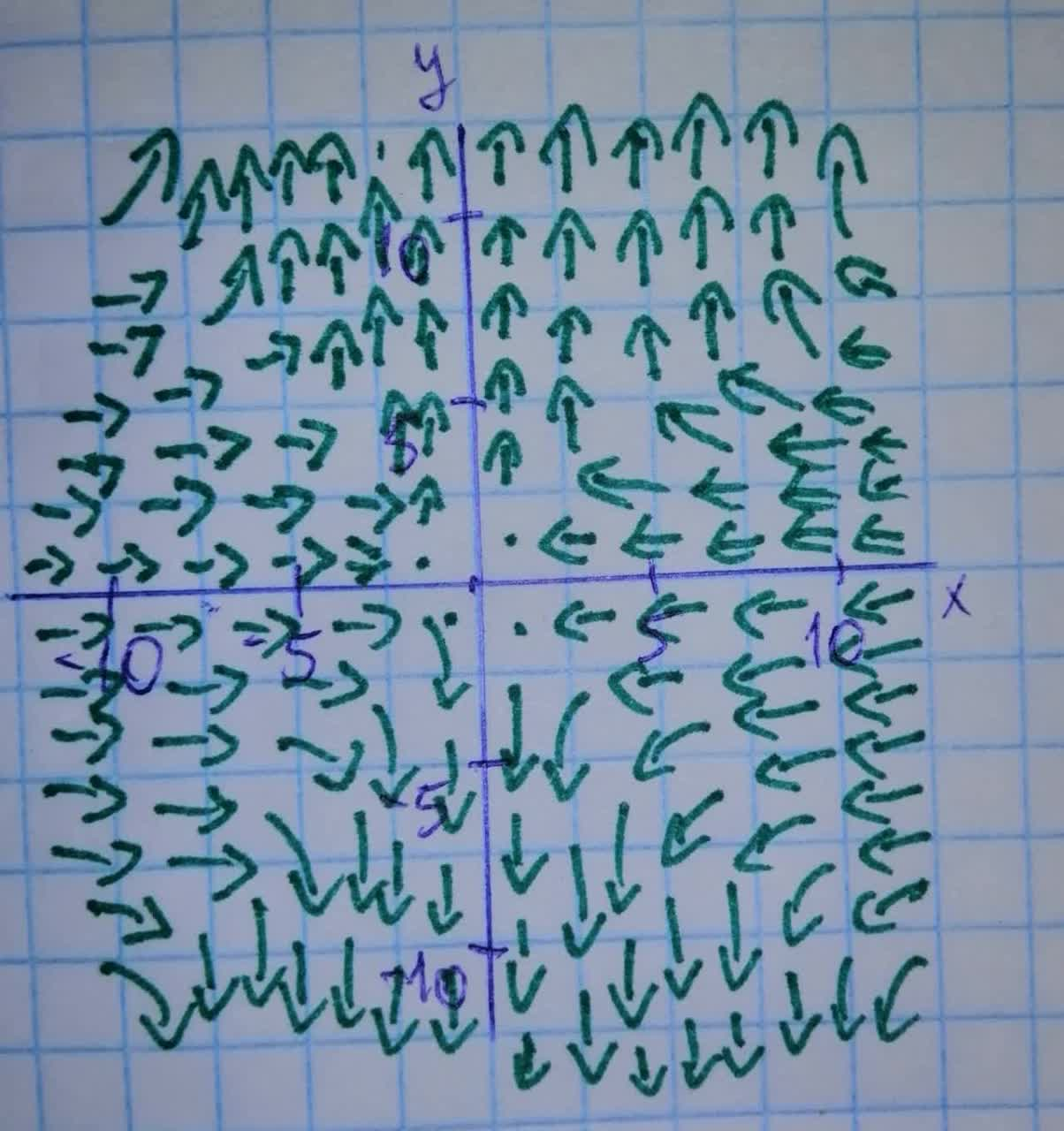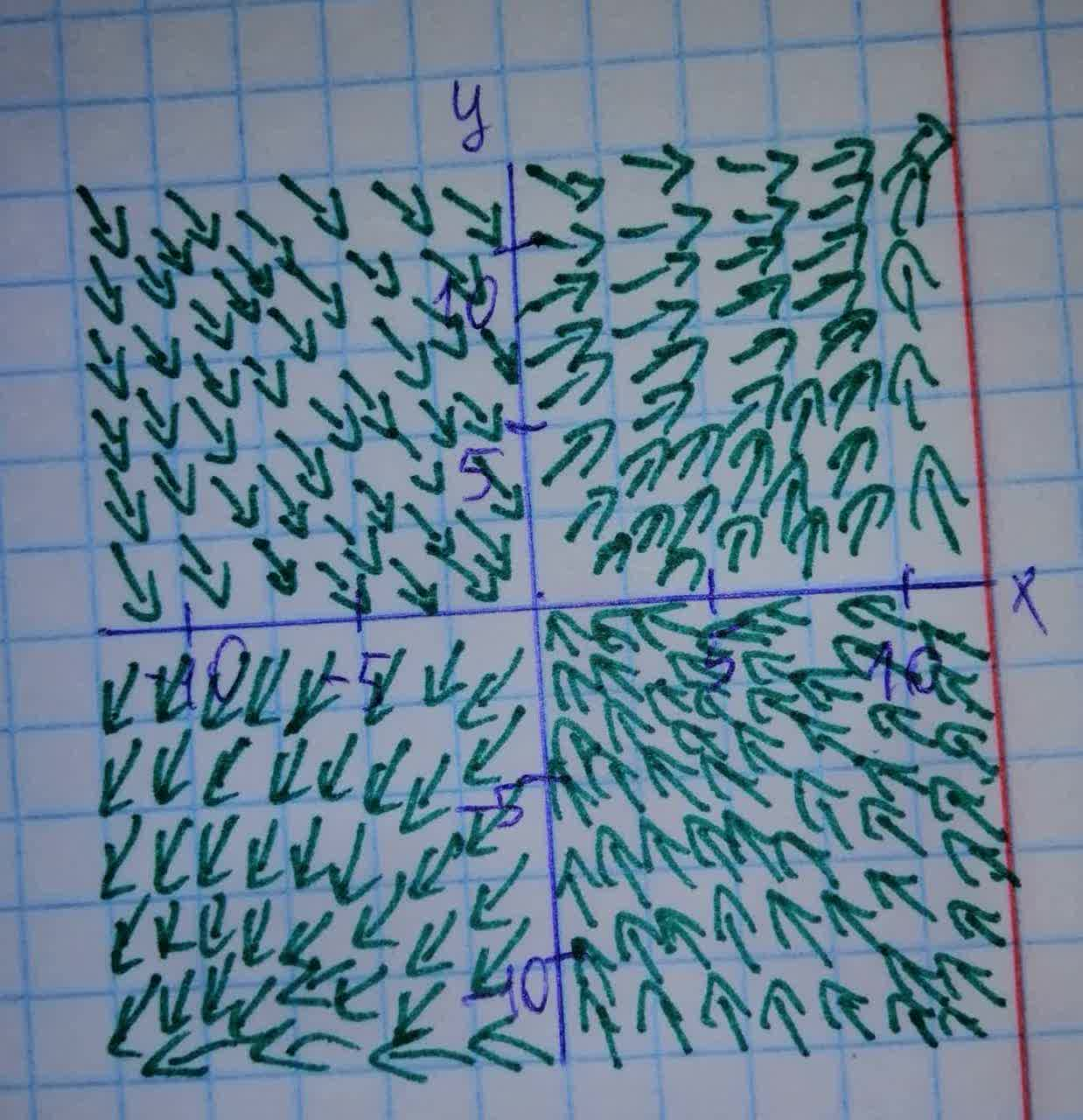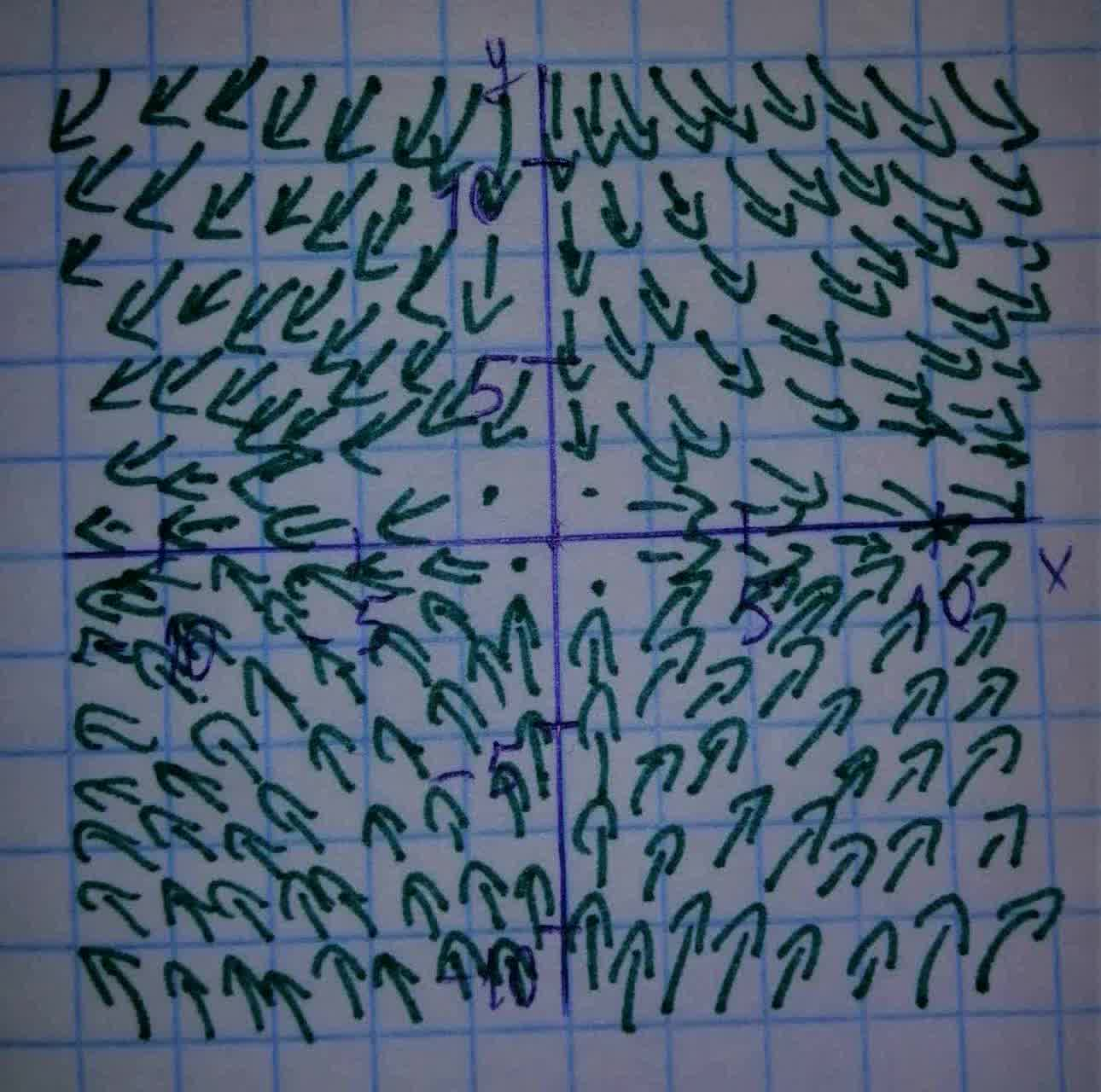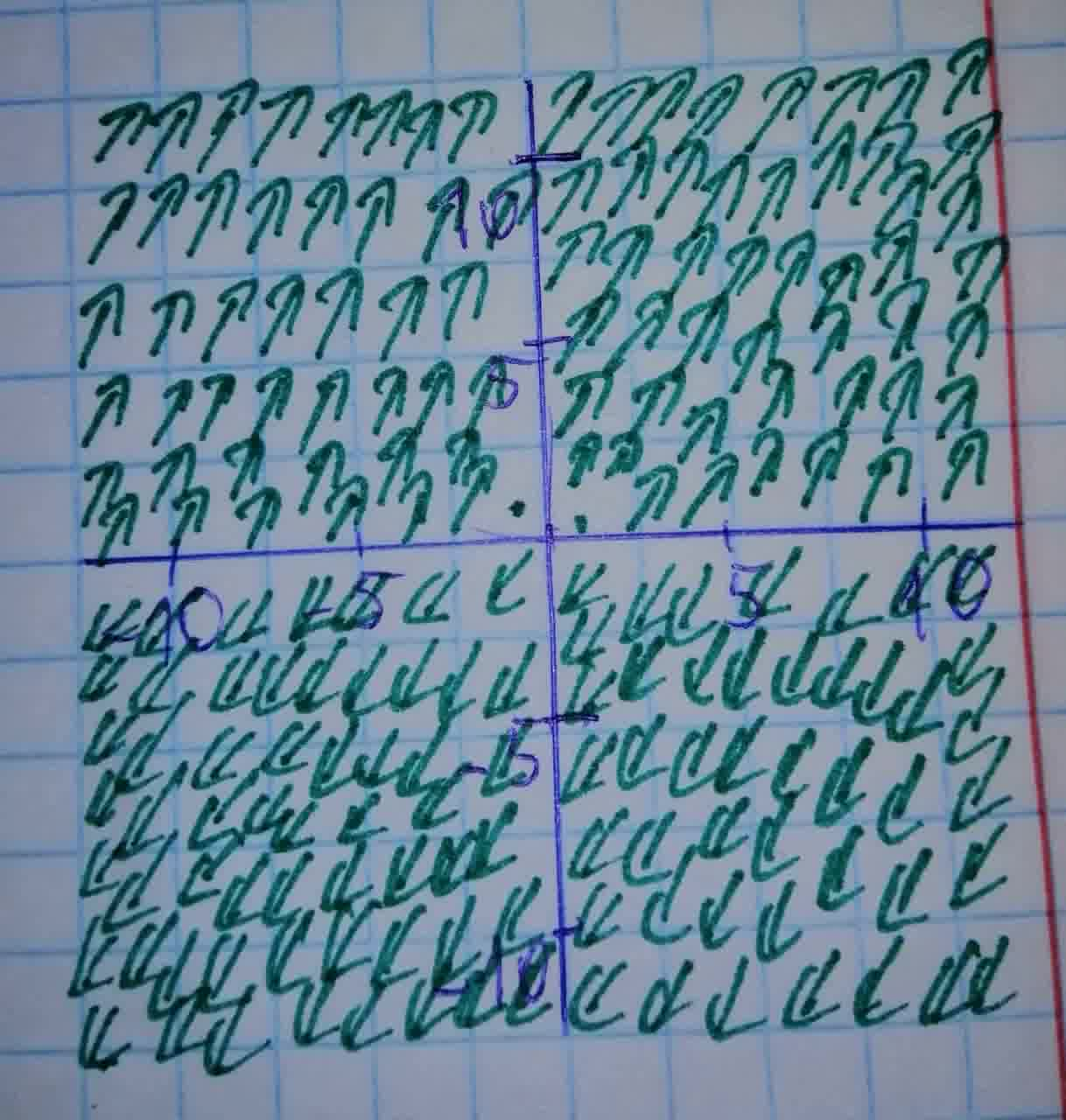• Questions are typically answered in as fast as 30 minutes

Solve your problem for the price of one coffee

• Math expert for every subject
• Pay only if we can solve itStephanie Mann

Step 1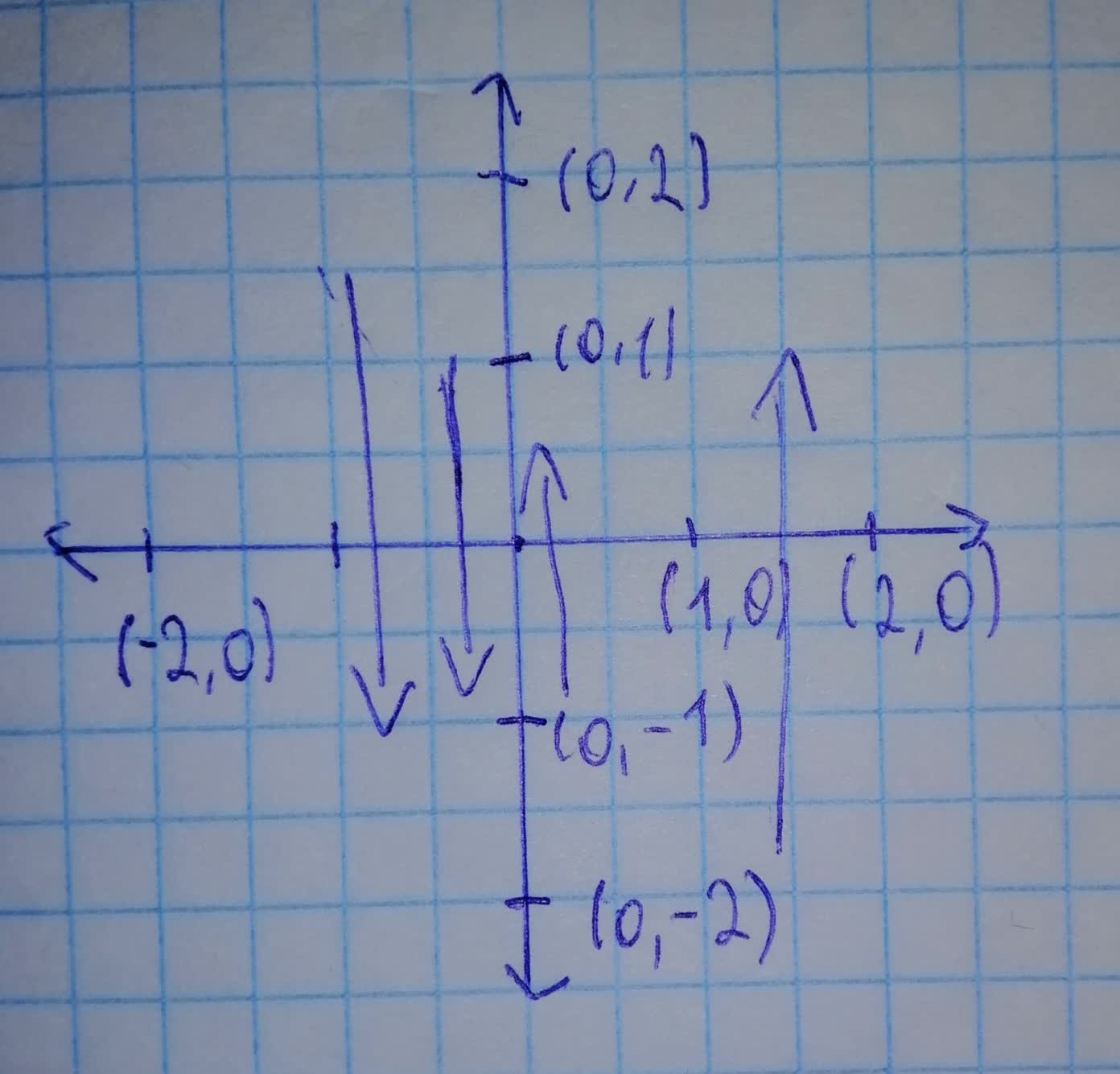$\begin{array}{|c|c|}\hline (x,\ y) & f=(x,\ -y) \\ \hline (1,\ 0) & (1,\ 0) \\ \hline (0,\ 1) & (0,\ -1) \\ \hline (-1,\ 0) & (-1,\ 0) \\ \hline (0,\ -1) & (0,\ 1) \\ \hline (2,\ 0) & (2,\ 0) \\ \hline (0,\ 2) & (0,\ -2) \\ \hline \end{array}$
Cleasy all points form first quadrant to all points of forte quadrant and vice versa.
Similars all point of d Second quadrant to all point of third quadrant vice verse.
Hence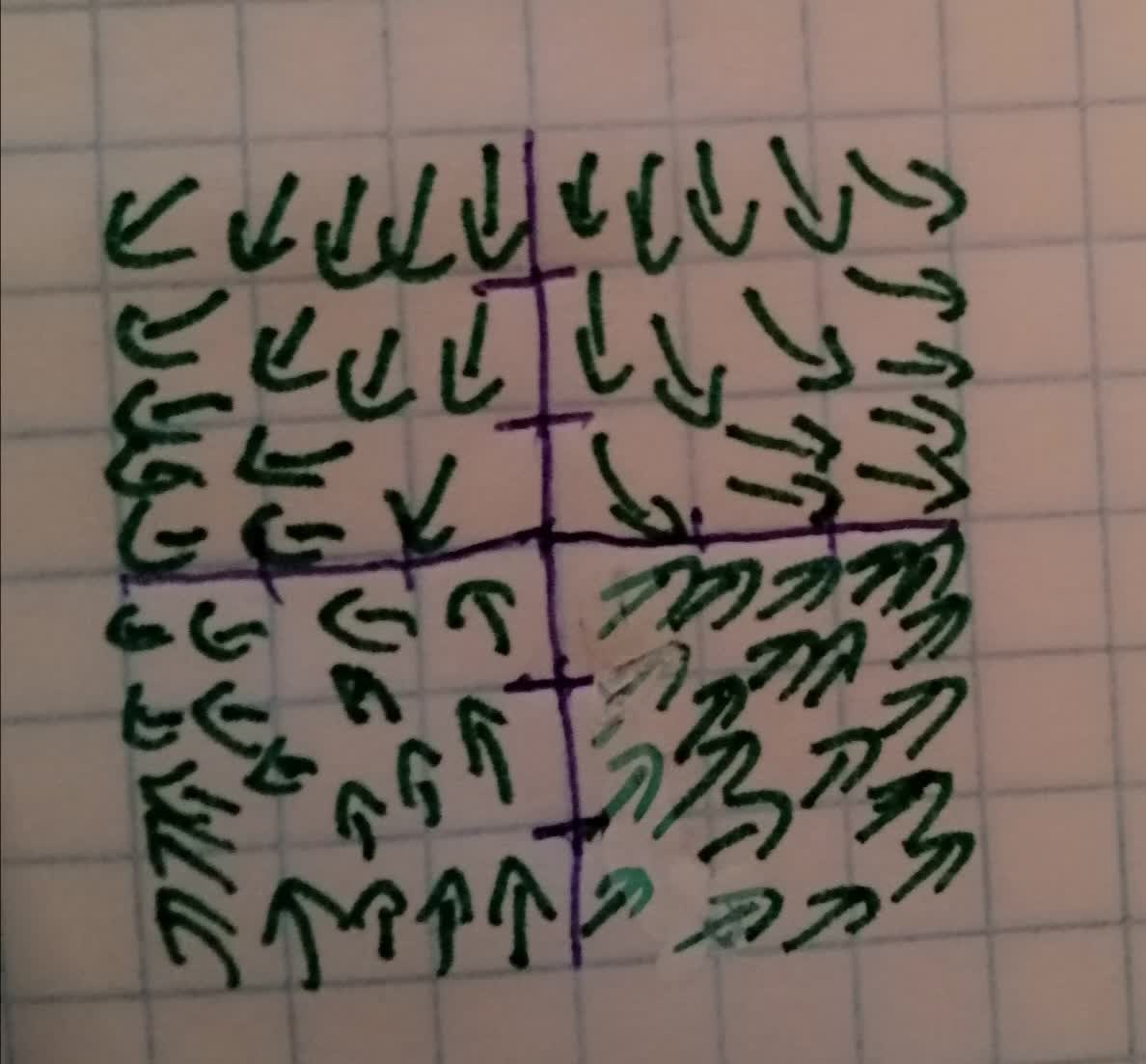third picture (That u lovert and left picture of the sween).